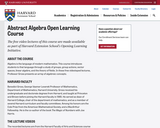Updating search results...

# 2 Results

View
Selected filters:
• Harvard UniversityRating
0.0 stars

Algebra is the language of modern mathematics. This course introduces students to that language through a study of groups, group actions, vector spaces, linear algebra, and the theory of fields.

Subject:
Algebra
Mathematics
Material Type:
Lecture
Provider:
Harvard University
Provider Set:
Harvard Extension School
Author:
Benedict Gross
01/22/2018Conditional Remix & Share Permitted
CC BY-NC-SA
Rating
0.0 stars

Some of the topics that this book addresses are: Vector spaces; finite-dimensional vector spaces; differential calculus; compactness and completeness; scalar product space; differential equations; multilenear functionals; integration; differentiable manifolds; integral calculus on manifolds; exterior calculus.

Note: this is a 57 MB PDF Document.

Subject:
Calculus
Mathematics
Material Type:
Textbook
Provider:
Harvard University
Provider Set:
Individual Authors
Author:
Sternberg Shlomo and Lynn Loomis